# A brief introduction to bibliometrix

#### Citation for package ‘bibliometrix’:

citation("bibliometrix")
##
## To cite bibliometrix in publications, please use:
##
##   Aria, M. and Cuccurullo C. (2016). bibliometrix: A R tool for
##   comprehensive bibliometric analysis of scientific literature.
##   Scientometrics, 1, pages 1-17.
##
## A BibTeX entry for LaTeX users is
##
##   @Article{,
##     title = {bibliometrix: A R tool for comprehensive bibliometric analysis of scientific literature},
##     author = {{Aria} and {Massimo} and {Cuccurullo} and {Corrado}},
##     journal = {Scientometrics},
##     volume = {1},
##     pages = {1--20},
##     publisher = {Springer},
##     year = {2016},
##   }

## Introduction

bibliometrix package provides a set of tools for quantitative research in bibliometrics and scientometrics.

Bibliometrics turns the main tool of science, quantitative analysis, on itself. Essentially, bibliometrics is the application of quantitative analysis and statistics to publications such as journal articles and their accompanying citation counts. Quantitative evaluation of publication and citation data is now used in almost all science fields to evaluate growth, maturity, leading authors, conceptual and intellectual maps, trend of a scientific community.

Bibliometrics is also used in research performance evaluation, especially in university and government labs, and also by policymakers, research directors and administrators, information specialists and librarians, and scholars themselves.

bibliometrix supports scholars in three key phases of analysis:

• Data importing and conversion to R format;

• Bibliometric analysis of a publication dataset;

• Building matrices for co-citation, coupling, and collaboration analysis. Matrices are the input data for performing network analysis or multidimensional scaling analysis.

## Bibliographic databases

bibliometrix works with data extracted from the two main bibliographic databases: SCOPUS and Thomson Reuter ISI Web of Knowledge.

SCOPUS (http://www.scopus.com), founded in 2004, offers a great deal of flexibility for the bibliometric user. It permits to query for different fields, such as titles, abstracts, keywords, references and so on. SCOPUS allows for relatively easy downloading data-queries, although there are some limits on very large results sets with over 2000 items.

Scopus is th more valuable resource for humanities.

ISI Web of Knowledge (WoK) (http://www.webofknowledge.com), owned by Thomson Reuter, was founded by Eugene Garfield, one of the pioneers of bibliometrics.
This platform includes many different collections, included the Web of Science Core Collection that covers social sciences and humanities.

## Data acquisition

Bibliographic data may be obtained by querying the SCOPUS or ISI WoK database by diverse fields within the database, such as topic, author, journal, timespan, and so on.

In this example, we show how to download data querying a keyword in the manuscript title field.

We choose the generic keyword “bibliometrics”.

### Querying from ISI WoK

At the link http://www.webofknowledge.com , select Web of Science Core Collection database.

Write the keyword “bibliometrics” in the search field and select title from the dropdown menu (see figure 1).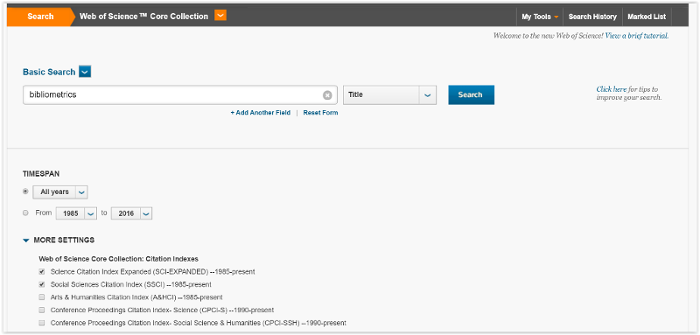Figure 1

Choose SCI-EXPANDED and SSCI citation indexes.

The search yielded 300 results on May 09, 2016.

Results can be refined using options on the left of the page (type of manuscript, sources, subject category, etc.).

After refining the query, you can add records to your Marked List by clicking the button “add to marked list” at the end of the page and selecting the records to save (see figure 2).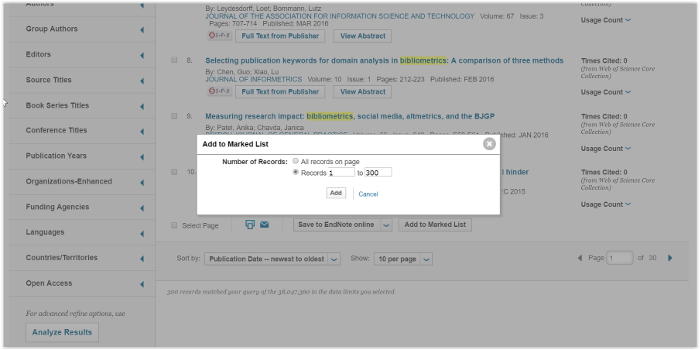Figure 2

The Marked List page provides you with a list of publications selected and various means of exporting data.

To export the data you desire, choose the export tool and follow the three intuitive steps (see figure 3).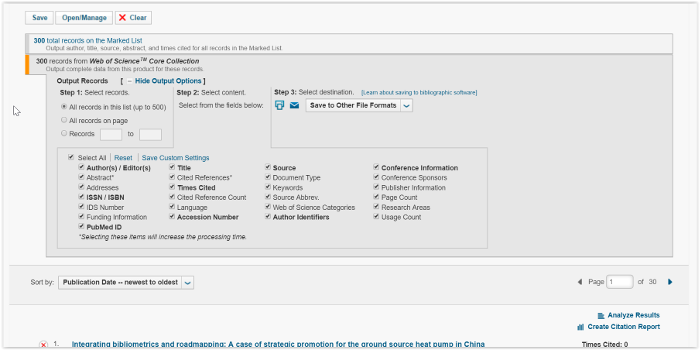Figure 3

The export tool allows you to select the diverse fields to save. So, select the fields your are interested in (for example all the available data about marked records).

To download an export file appropriate for bibliometrix package, make sure to select the format option “Save to Other File Formats” and choose Bibtex or Plain Text.

Please pay attention that bibtex import function is faster than plain text.

The ISI platform permits to export only 500 records at a time. So you will have to manually combine data sets after downloading all publications.

The ISI Web of Science export tool creates an export file with a default name “savedrecs” with extention “.txt” or “.bib” for plain text or bibtex format respectively.

### Querying from SCOPUS

To find all articles whose title include the terms “bibliometrics” simply write this keyword in the field and select “Article Title” (see figure 4)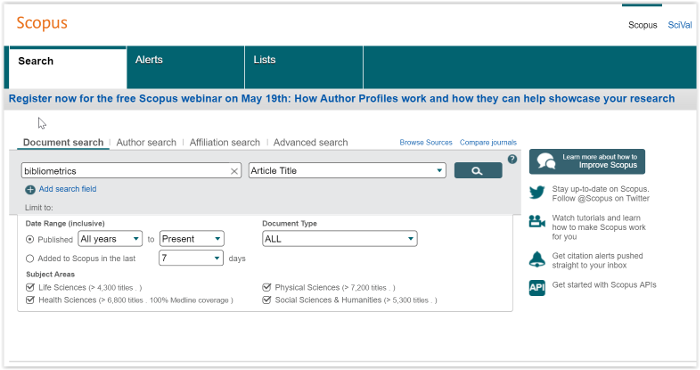Figure 4

The search yielded 414 results on May 09, 2016.

You can download the references (up to 2,000 full records) by checking the ‘Select All’ box and clicking on the link ‘Export’. Choose the file type “bibtex export” and “all available information” (see figure 5).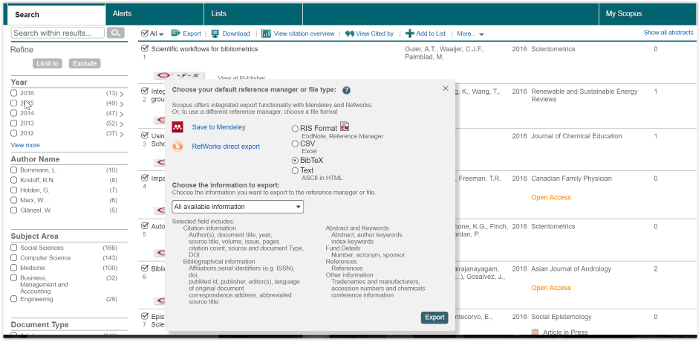Figure 5

The SCOPUS export tool creates an export file with the default name “scopus.bib”.

## Data preparation

To assure that the export file will be compatible with R, you need to modify it with a text editor.

First, make sure to delete the “EF” (End File) tag that closes the ISI file. Second, change the file format to “UTF-8 codify without BOM” because the file have to be saved without Byte order mark (U+FEFF) at the beginning.

The export file can be read by R using the function readLines:

D <- readLines("http://www.bibliometrix.org/datasets/savedrecs.bib")

D is a large character object.

It can be converted in a data frame using the function convert2df:

library(bibliometrix)
##
## bibliometrix
## A R tool for comprehensive bibliometric analysis of scientific literature
##
## by Massimo Aria & Corrado Cuccurullo
##
## http:\\www.bibliometrix.org
M <- convert2df(D, dbsource = "isi", format = "bibtex")
## Articles extracted   100
## Articles extracted   200
## Articles extracted   300

convert2df creates a bibliographic data frame with cases corresponding to manuscripts and variables to Field Tag in the original export file.

Each manuscript contains several elements, such as authors’ names, title, keywords and other information. All these elements constitute the bibliographic attributes of a document, also called metadata.

Data frame columns are named using the standard ISI WoS Field Tag codify.

The main field tags are:

Field Tag Description
AU Authors
TI Document Title
SO Publication Name (or Source)
JI ISO Source Abbreviation
DT Document Type
DE Authors’ Keywords
ID Keywords associated by SCOPUS or ISI database
AB Abstract
CR Cited References
TC Times Cited
PY Year
SC Subject Category
UT Unique Article Identifier
DB Bibliographic Database

## Bibliometric Analysis

The first step is to perform a descriptive analysis of the bibliographic data frame.

The function biblioAnalysis calculates main bibliometric measures using simply the syntax:

results <- biblioAnalysis(M, sep = ";")

The function biblioAnalysis returns an object of class “bibliometrix”.

An object of class “bibliometrix” is a list containing the following components:

List element Description
Articles the total number of manuscripts
Authors the authors’ frequency distribution
AuthorsFrac the authors’ frequency distribution (fractionalized)
FirstAuthors first author of each manuscript
nAUperPaper the number of authors per manuscript
Apparences the number of author apparences
nAuthors the number of authors
AuMultiAuthoredArt the number of authors of multi authored articles
Years pubblication year of each manuscript
FirstAffiliation the affiliation of the first author
Affiliations the frequency distribution of affiliations (of all co-authors for each paper)
Aff_frac the fractionalized frequency distribution of affiliations (of all co-authors for each paper)
CO the affiliation country of first author
Countries the affiliation countries’ frequency distribution
TotalCitation the number of times each manuscript has been cited
TCperYear the yearly average number of times each manuscript has been cited
Sources the frequency distribution of sources (journals, books, etc.)
DE the frequency distribution of authors’ keywords
ID the frequency distribution of keywords associated to the manuscript by SCOPUS and Thomson Reuters’ ISI Web of Knowledge databases

### Functions summary and plot

To summarize main results of the bibliometric analysis, use the generic function summary. It displays main information about the bibliographic data frame and 6 tables: annual scientific production, most productive authors, most productive countries, total citation per country, most relevant sources (journals) and most relevant keywords.

summary accepts two additional arguments. k is a formatting value that indicates the number of rows of each table. pause is a logical value (TRUE or FALSE) used to allow (or not) pause in screen scrolling. Choosing k=10 you decide to see the first 10 Authors, the first 10 sources, etc.

S=summary(object = results, k = 10, pause = FALSE)
##
##
##
##  Articles                              300
##  Sources (Journals, Books, etc.)       144
##  Keywords Plus (ID)                    488
##  Author's Keywords (DE)                383
##  Period                                1985 - 2016
##  Average citations per article         11.39
##
##  Authors                               582
##  Author Appearances                    689
##  Authors of single authored articles   110
##  Authors of multi authored articles    472
##
##  Articles per Author                   0.515
##  Authors per Article                   1.94
##  Co-Authors per Articles               2.3
##  Collaboration Index                   3.05
##
##
## Annual Scientific Production
##
##  Year    Articles
##     1985        4
##     1986        3
##     1987        6
##     1988        7
##     1989        8
##     1990        6
##     1991        7
##     1992        6
##     1993        5
##     1994        7
##     1995        1
##     1996        8
##     1997        4
##     1998        5
##     1999        2
##     2000        7
##     2001        8
##     2002        5
##     2003        1
##     2004        3
##     2005       12
##     2006        5
##     2007        5
##     2008        8
##     2009       14
##     2010       17
##     2011       20
##     2012       25
##     2013       21
##     2014       29
##     2015       32
##     2016        9
##
## Annual Percentage Growth Rate 2.650419
##
##
## Most Productive Authors
##
##            Authors        Articles Authors        Articles Fractionalized
## 1  BORNMANN,LUTZ                 9 BORNMANN,LUTZ                     5.17
## 2  KOSTOFF,RN                    8 MARX,WERNER                       3.17
## 3  MARX,WERNER                   6 ATKINSON,ROGER                    3.00
## 4  HUMENIK,JA                    5 BROADUS,RN                        3.00
## 5  ABRAMO,GIOVANNI               4 CRONIN,B                          3.00
## 6  D'ANGELO,CIRIACOANDREA        4 BORGMAN,CL                        2.50
## 7  GLANZEL,W                     4 MCCAIN,KW                         2.50
## 8  ATKINSON,ROGER                3 PERITZ,BC                         2.50
## 9  BARKER,K                      3 KOSTOFF,RN                        2.10
## 10 BORGMAN,CL                    3 ADAMS,JONATHAN                    2.00
##
##
## Most Productive Countries
##
##    Country   Articles   Freq
## 1  USA             84 0.3043
## 2  ENGLAND         27 0.0978
## 3  GERMANY         17 0.0616
## 4  FRANCE          13 0.0471
## 5  BRAZIL          12 0.0435
## 6  CHINA           12 0.0435
## 8  INDIA           10 0.0362
## 9  SPAIN            9 0.0326
## 10 AUSTRALIA        8 0.0290
##
##
## Total Citations per Country
##
##    Country      Total Citations Average Article Citations
## 1  USA                     1834                     21.83
## 2  GERMANY                  330                     19.41
## 3  ITALY                    163                     32.60
## 4  AUSTRALIA                134                     16.75
## 5  ENGLAND                  121                      4.48
## 7  INDIA                     85                      8.50
## 8  SPAIN                     85                      9.44
## 9  IRAN                      74                     37.00
## 10 BELGIUM                   70                     10.00
##
##
## Most Relevant Sources
##
##                                                            Sources
## 1  SCIENTOMETRICS
## 2  JOURNAL OF THE AMERICAN SOCIETY FOR INFORMATION SCIENCE AND TECHNOLOGY
## 3  JOURNAL OF THE AMERICAN SOCIETY FOR INFORMATION SCIENCE
## 4  JOURNAL OF INFORMETRICS
## 5  JOURNAL OF DOCUMENTATION
## 6  JOURNAL OF INFORMATION SCIENCE
## 7  BRITISH JOURNAL OF ANAESTHESIA
## 8  LIBRI
## 9  SOCIAL WORK IN HEALTH CARE
## 10 TECHNOLOGICAL FORECASTING AND SOCIAL CHANGE
##    Articles
## 1        49
## 2        14
## 3         8
## 4         7
## 5         6
## 6         6
## 7         5
## 8         5
## 9         5
## 10        5
##
##
## Most Relevant Keywords
##
##    Author Keywords (DE)      Articles Keywords-Plus (ID)     Articles
## 1      BIBLIOMETRICS               65    SCIENCE                   41
## 2      CITATION ANALYSIS           11    INDICATORS                26
## 3      SCIENTOMETRICS               7    IMPACT                    23
## 4      H-INDEX                      5    CITATION                  20
## 5      IMPACT FACTOR                5    CITATION ANALYSIS         16
## 6      INFORMATION RETRIEVAL        5    JOURNALS                  15
## 7      PEER REVIEW                  5    H-INDEX                   14
## 8      ANALYSIS                     4    PUBLICATION               12
## 9      CITATION                     4    INFORMATION-SCIENCE       10
## 10     CITATIONS                    4    IMPACT FACTORS             8

Some basic plots can be drawn using the generic function :

plot(x = results, k = 10, pause = FALSE)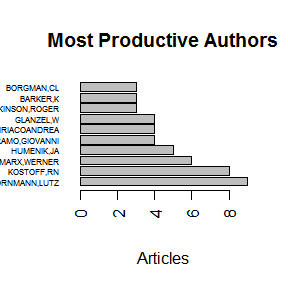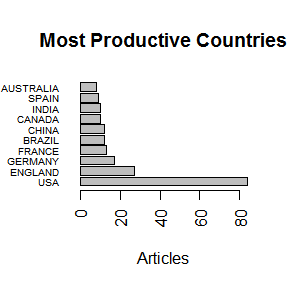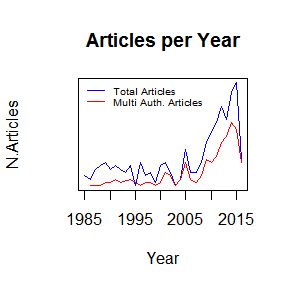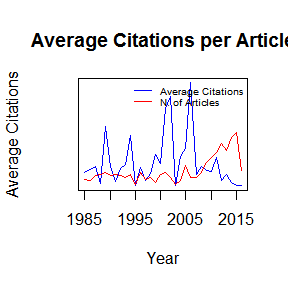## Analysis of Cited References

The function citations generates the frequency table of the most cited references or the most cited first authors (of references).

For each manuscript, cited references are in a single string stored in the column “CR” of the data frame.

For a correct extraction, you need to identify the separator field among different references, used by ISI or SCOPUS database. Usually, the default separator is “;” or ". " (a dot with double space).

# M$CR The figure shows the reference string of the first manuscript. In this case, the separator field is sep = ". ".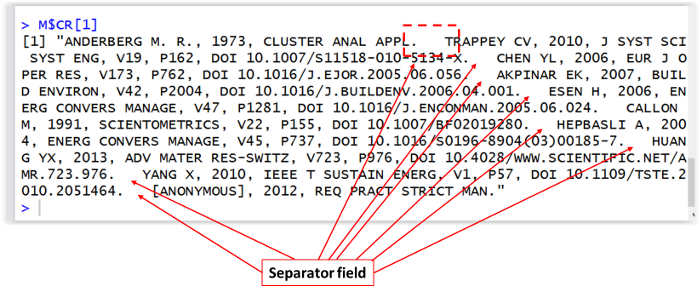Figure 6 To obtain the most frequent cited manuscripts: CR <- citations(M, field = "article", sep = ". ") CR$Cited[1:10]
## CR
## HIRSCH JE, 2005, P NATL ACAD SCI USA, V102, P16569, DOI 10.1073/PNAS.0507655102
##                                                                              29
##       SMALL H, 1973, J AM SOC INFORM SCI, V24, P265, DOI 10.1002/ASI.4630240406
##                                                                              19
##                                     DE SOLLA PRICE DJ, 1963, LITTLE SCI BIG SCI
##                                                                              15
##                              BRADFORD S. C, 1934, ENGINEERING-LONDON, V137, P85
##                                                                              14
##                                              PRITCHAR.A, 1969, J DOC, V25, P348
##                                                                              14
##     GARFIELD E, 2006, JAMA-J AM MED ASSOC, V295, P90, DOI 10.1001/JAMA.295.1.90
##                                                                              11
##                                     COLE FRANCIS J., 1917, SCI PROGR, V11, P578
##                                                                              10
##         EGGHE L, 2006, SCIENTOMETRICS, V69, P131, DOI 10.1007/S11192-006-0144-7
##                                                                              10
##                  KESSLER MM, 1963, AM DOC, V14, P10, DOI 10.1002/ASI.5090140103
##                                                                              10
##          SMALL HG, 1978, SOC STUD SCI, V8, P327, DOI 10.1177/030631277800800305
##                                                                              10

To obtain the most frequent cited first authors:

CR <- citations(M, field = "author", sep = ".  ")
CR$Cited[1:10] ## CR ## GARFIELD E BORNMANN L SMALL H WHITE HD CRONIN B ## 107 70 61 47 46 ## KOSTOFF RN LEYDESDORFF L GLANZEL W NARIN F BROOKES BC ## 46 46 44 40 38 The function localCitations generates the frequency table of the most local cited authors. Local citations measure how many times an author included in this collection have been cited by other authors also in the collection. To obtain the most frequent local cited authors: CR <- localCitations(M, results, sep = ". ") CR[1:10] ## CR ## WHITE HD CRONIN B KOSTOFF RN GLANZEL W NARIN F BROOKES BC ## 47 46 46 44 40 38 ## SCHUBERT A MCCAIN KW SENGUPTA IN LINE MB ## 37 25 23 21 ## Authors’ Dominance ranking The function dominance calculates the authors’ dominance ranking as proposed by Kumar & Kumar, 2008. Function arguments are: results (object of class bibliometrix) obtained by biblioAnalysis; and k (the number of authors to consider in the analysis). DF <- dominance(results, k = 10) DF ## Dominance Factor Multi Authored First Authored ## KOSTOFF,RN 1.0000000 8 8 ## HOLDEN,G 1.0000000 3 3 ## ABRAMO,GIOVANNI 0.7500000 4 3 ## GLANZEL,W 0.7500000 4 3 ## GARG,KC 0.6666667 3 2 ## MOPPETT,IK 0.6666667 3 2 ## BORNMANN,LUTZ 0.5555556 9 5 ## BORGMAN,CL 0.3333333 3 1 ## D'ANGELO,CIRIACOANDREA 0.2500000 4 1 ## MARX,WERNER 0.1666667 6 1 ## Rank by Articles Rank by DF ## KOSTOFF,RN 2 1 ## HOLDEN,G 9 2 ## ABRAMO,GIOVANNI 4 3 ## GLANZEL,W 6 4 ## GARG,KC 8 5 ## MOPPETT,IK 10 6 ## BORNMANN,LUTZ 1 7 ## BORGMAN,CL 7 8 ## D'ANGELO,CIRIACOANDREA 5 9 ## MARX,WERNER 3 10 The Dominance Factor is a ratio indicating the fraction of multi authored articles in which a scholar appears as first author. In this example, Kostoff and Holden dominate their research team because they appear as first authors in all their papers (8 for Kostoff and 3 for Holden). ## Authors’ h-index The h-index is an author-level metric that attempts to measure both the productivity and citation impact of the publications of a scientist or scholar. The index is based on the set of the scientist’s most cited papers and the number of citations that they have received in other publications. The function Hindex calculates the authors’ H-index and its variants (g-index and m-index) in a bibliographic collection. Function arguments are: M a bibliographic data frame; auhtors a character vector containing the the authors’ names for which you want to calculate the H-index. The aurgument has the form C(“SURNAME1 N”,“SURNAME2 N”,…). In other words, for each author: surname and initials are separated by one blank space. i.e for the authors SEMPRONIO TIZIO CAIO and ARIA MASSIMO authors argument is authors = c(“SEMPRONIO TC”, “ARIA M”). To calculate the h-index of Lutz Bornmann in this collection: indices <- Hindex(M, authors="BORNMANN L", sep = ";") # Bornmann's impact indices: indices$H
##       Author h_index g_index m_index
## 1 BORNMANN L       4       7     0.8
# Bornmann's citations
indices$CitationList ## [] ## Authors Journal Year ## 1 LEYDESDORFF LOET ;BORNMANN LUT JOURNAL OF THE ASSOCIATION FOR 2016 ## 2 BORNMANN LUTZ ;MARX WERNER JOURNAL OF INFORMETRICS 2015 ## 3 MARX WERNER ;BORNMANN LUTZ SOZIALE WELT-ZEITSCHRIFT FUR S 2015 ## 4 BORNMANN LUTZ RESEARCH EVALUATION 2014 ## 5 BORNMANN LUTZ ;LEYDESDORFF LOE JOURNAL OF INFORMETRICS 2014 ## 6 BORNMANN LUTZ JOURNAL OF THE AMERICAN SOCIET 2013 ## 7 BORNMANN LUTZ ;WILLIAMS RICHAR JOURNAL OF INFORMETRICS 2013 ## 8 BORNMANN LUTZ ;MARX WERNER JOURNAL OF INFORMETRICS 2013 ## 9 BORNMANN LUTZ ;BOWMAN BENJAMIN ZEITSCHRIFT FUR EVALUATION 2012 ## TotalCitation ## 1 0 ## 2 1 ## 3 1 ## 4 2 ## 5 3 ## 6 5 ## 7 10 ## 8 11 ## 9 18 To calculate the h-index of the first 10 most productive authors (in this collection): authors=gsub(","," ",names(results$Authors)[1:10])

indices <- Hindex(M, authors, sep = ";")

indices$H ## Author h_index g_index m_index ## 1 BORNMANN LUTZ 4 7 0.80000000 ## 2 KOSTOFF RN 8 8 0.44444444 ## 3 MARX WERNER 3 6 0.50000000 ## 4 HUMENIK JA 5 5 0.29411765 ## 5 ABRAMO GIOVANNI 4 4 0.50000000 ## 6 D'ANGELO CIRIACOANDREA 4 4 0.50000000 ## 7 GLANZEL W 2 5 0.08695652 ## 8 ATKINSON ROGER 0 0 0.00000000 ## 9 BARKER K 3 3 0.25000000 ## 10 BORGMAN CL 3 3 0.10714286 ## Lotka’s Law coefficient estimation The function lotka estimates Lotka’s law coefficients for scientific productivity (Lotka A.J., 1926). Lotka’s law describes the frequency of publication by authors in any given field as an inverse square law, where the number of authors publishing a certain number of articles is a fixed ratio to the number of authors publishing a single article. This assumption implies that the theoretical beta coefficient of Lotka’s law is equal to 2. Using lotka function is possible to estimate the Beta coefficient of our bibliographic collection and assess, through a statistical test, the similarity of this empirical distribution with the theoretical one. L <- lotka(results) # Author Productivity. Empirical Distribution L$AuthorProd
##   N.Articles N.Authors        Freq
## 1          1       515 0.884879725
## 2          2        46 0.079037801
## 3          3        14 0.024054983
## 4          4         3 0.005154639
## 5          5         1 0.001718213
## 6          6         1 0.001718213
## 7          8         1 0.001718213
## 8          9         1 0.001718213
# Beta coefficient estimate
L$Beta ##  3.04525 # Constant L$C
##  0.6018257
# Goodness of fit
L$R2 ##  0.9353053 # P-value of K-S two sample test L$p.value
##  0.08786641

The table L$AuthorProd shows the observed distribution of scientific productivity in our example. The estimated Beta coefficient is 3.05 with a goodness of fit equal to 0.94. Kolmogorov-Smirnoff two sample test provides a p-value 0.09 that means there is not a significant difference between the observed and the theoretical Lotka distributions. You can compare the two distributions using plot function: # Observed distribution Observed=L$AuthorProd[,3]

# Theoretical distribution with Beta = 2
Theoretical=10^(log10(L$C)-2*log10(L$AuthorProd[,1]))

plot(L$AuthorProd[,1],Theoretical,type="l",col="red",ylim=c(0, 1), xlab="Articles",ylab="Freq. of Authors",main="Scientific Productivity") lines(L$AuthorProd[,1],Observed,col="blue")
legend(x="topright",c("Theoretical (B=2)","Observed"),col=c("red","blue"),lty = c(1,1,1),cex=0.6,bty="n")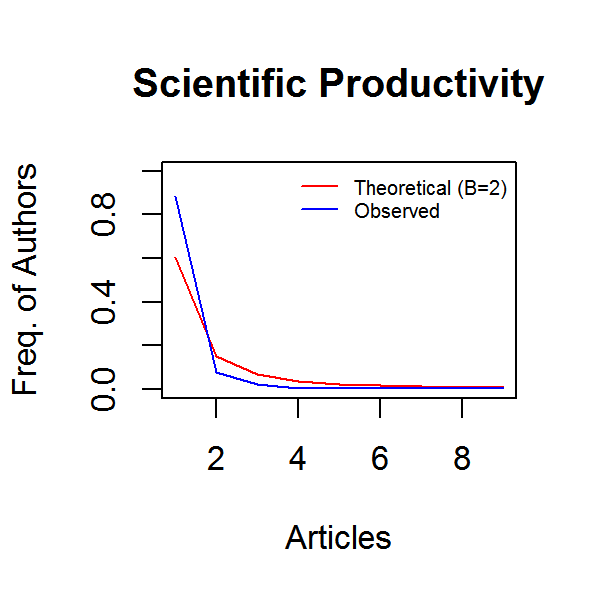## Bibliometric network matrices

Manuscript’s attributes are connected to each other through the manuscript itself: author(s) to journal, keywords to publication date, etc.

These connections of different attributes generate bipartite networks that can be represented as rectangular matrices (Manuscripts x Attributes).

Furthermore, scientific publications regularly contain references to other scientific works. This generates a further network, namely, co-citation or coupling network.

These networks are analysed in order to capture meaningful properties of the underlying research system, and in particular to determine the influence of bibliometric units such as scholars and journals.

### Bipartite networks

cocMatrix is a general function to compute a bipartite network selecting one of the metadata attributes.

For example, to create a network Manuscript x Publication Source you have to use the field tag “SO”:

A <- cocMatrix(M, Field = "SO", sep = ";")

A is a rectangular binary matrix, representing a bipartite network where rows and columns are manuscripts and sources respectively.

The generic element $$a_{ij}$$ is 1 if the manuscript $$i$$ has been published in source $$j$$, 0 otherwise.

The $$j-th$$ column sum $$a_j$$ is the number of manuscripts published in source $$j$$.

Sorting, in decreasing order, the column sums of A, you can see the most relevant publication sources:

sort(Matrix::colSums(A), decreasing = TRUE)[1:5]
##                                                         SCIENTOMETRICS
##                                                                     49
## JOURNAL OF THE AMERICAN SOCIETY FOR INFORMATION SCIENCE AND TECHNOLOGY
##                                                                     14
##                JOURNAL OF THE AMERICAN SOCIETY FOR INFORMATION SCIENCE
##                                                                      8
##                                                JOURNAL OF INFORMETRICS
##                                                                      7
##                                               JOURNAL OF DOCUMENTATION
##                                                                      6

Following this approach, you can compute several bipartite networks:

• Citation network
# A <- cocMatrix(M, Field = "CR", sep = ".  ")
• Author network
# A <- cocMatrix(M, Field = "AU", sep = ";")
• Country network

Authors’ Countries is not a standard attribute of the bibliographic data frame. You need to extract this information from affiliation attribute using the function metaTagExtraction.

M <- metaTagExtraction(M, Field = "AU_CO", sep = ";")
# A <- cocMatrix(M, Field = "AU_CO", sep = ";")

metaTagExtraction allows to extract the following additional field tags: Authors’ countries (Field = "AU_CO"); First author of each cited reference (Field = "CR_AU"); and Publication source of each cited reference (Field = "CR_SO").

• Author keyword network
# A <- cocMatrix(M, Field = "DE", sep = ";")
• Keyword Plus network
# A <- cocMatrix(M, Field = "ID", sep = ";")
• Etc.

### Bibliographic coupling

Two articles are said to be bibliographically coupled if at least one cited source appears in the bibliographies or reference lists of both articles (Kessler, 1963).

A coupling network can be obtained using the general formulation:

$B = A \times A^T$ where A is a bipartite network.

Element $$b_{ij}$$ indicates how many bibliographic coupling exist between manuscripts $$i$$ and $$j$$. In other words, $$b_{ij}$$ gives the number of paths of length 2, via which one moves from $$i$$ along the arrow and then to $$j$$ in the opposite direction.

$$B$$ is a simmetrical matrix $$B = B^T$$.

The strength of the coupling of two articles, $$i$$ and $$j$$ is defined simply by the number of references that the articles have in common, as given by the element $$b_{ij}$$ of matrix $$B$$.

The function biblioNetwork calculates, starting from a bibliographic data frame, the most frequently used coupling networks: Authors, Sources, Keywords and Countries.

biblioNetwork uses two arguments to define the network to compute:

• analysis argument can be “collaboration”, “coupling” or “co-citation”

• network argument can be “authors”, “references”, “sources”, “countries”,“keywords” or “author_keywords”

The following code calculates a classical article coupling network:

# NetMatrix <- biblioNetwork(M, analysis = "coupling", network = "references", sep = ".  ")

Articles with only a few references, therefore, would tend to be more weakly bibliographically coupled, if coupling strength is measured simply according to the number of references articles contain in common.

This suggests that it might be more practicable to switch to a relative measure of bibliographic coupling.

couplingSimilarity function calculates Jaccard or Salton similarity coefficient among manuscripts of a coupling network.

NetMatrix <- biblioNetwork(M, analysis = "coupling", network = "sources", sep = ";")

# calculate jaccard similarity coefficient
S <- couplingSimilarity(NetMatrix, type="jaccard")

# plot journals' similarity (with min 3 manuscripts)
diag <- Matrix::diag
MapDegree <- 3
NETMAP <- S[diag(NetMatrix)>=MapDegree,diag(NetMatrix)>=MapDegree]
diag(NETMAP) <- 0

H <- heatmap(max(NETMAP)-as.matrix(NETMAP),symm=T, cexRow=0.3,cexCol=0.3)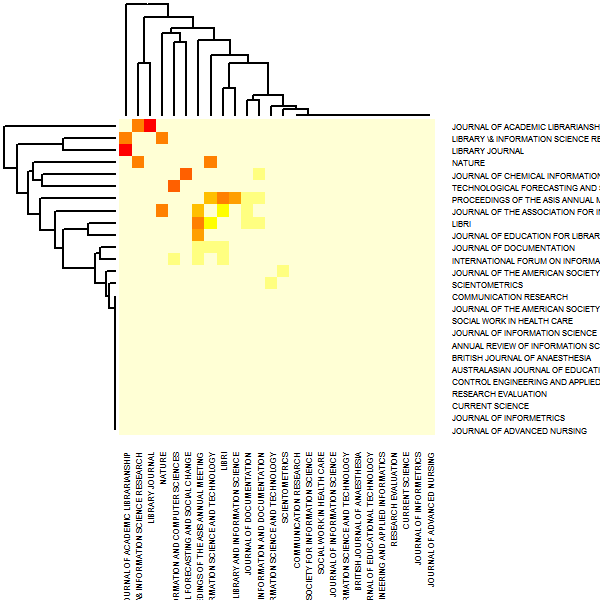### Bibliographic co-citation

We talk about co-citation of two articles when both are cited in a third article. Thus, co-citation can be seen as the counterpart of bibliographic coupling.

A co-citation network can be obtained using the general formulation:

$C = A^T \times A$ where A is a bipartite network.

Like matrix $$B$$, matrix $$C$$ is also symmetric. The main diagonal of $$C$$ contains the number of cases in which a reference is cited in our data frame.

In other words, the diagonal element $$c_{i}$$ is the number of local citations of the reference $$i$$.

Using the function biblioNetwork, you can calculate a classical reference co-citation network:

# NetMatrix <- biblioNetwork(M, analysis = "co-citation", network = "references", sep = ".  ")

### Bibliographic collaboration

Scientific collaboration network is a network where nodes are authors and links are co-authorships as the latter is one of the most well documented forms of scientific collaboration (Glanzel, 2004).

An author collaboration network can be obtained using the general formulation:

$AC = A^T \times A$ where A is a bipartite network Manuscripts x Authors.

The diagonal element $$ac_{i}$$ is the number of manuscripts authored or co-authored by researcher $$i$$.

Using the function biblioNetwork, you can calculate an authors’ collaboration network:

# NetMatrix <- biblioNetwork(M, analysis = "collaboration", network = "authors", sep = ";")

or a country collaboration network:

# NetMatrix <- biblioNetwork(M, analysis = "collaboration", network = "countries", sep = ";")

### Visualizing bibliographic networks

All bibliographic networks can be graphically visualized or modeled.

Here, we show how to visualize networks using package igraph.

# Load package igraph (install if needed)

require(igraph)
## Loading required package: igraph
##
## Attaching package: 'igraph'
## The following objects are masked from 'package:stats':
##
##     decompose, spectrum
## The following object is masked from 'package:base':
##
##     union

### Country Scientific Collaboration

# Create a country collaboration network

M <- metaTagExtraction(M, Field = "AU_CO", sep = ";")
NetMatrix <- biblioNetwork(M, analysis = "collaboration", network = "countries", sep = ";")

# define functions from package Matrix
diag <- Matrix::diag
colSums <-Matrix::colSums

ind <- which(Matrix::colSums(NetMatrix)-Matrix::diag(NetMatrix)>0)
NET <- NetMatrix[ind,ind]

# Select number of vertices to plot
n <- 20    # n. of vertices
NetDegree <- sort(diag(NET),decreasing=TRUE)[n]
NET <- NET[diag(NET)>=NetDegree,diag(NET)>=NetDegree]

# delete diagonal elements (self-loops)
diag(NET) <- 0

# Create igraph object

# Compute node degrees (#links) and use that to set node size:
deg <- degree(bsk.network, mode="all")
V(bsk.network)$size <- deg*1.1 # Remove loops bsk.network <- simplify(bsk.network, remove.multiple = F, remove.loops = T) # Choose Network layout #l <- layout.fruchterman.reingold(bsk.network) l <- layout.circle(bsk.network) #l <- layout.sphere(bsk.network) #l <- layout.mds(bsk.network) #l <- layout.kamada.kawai(bsk.network) ## Plot the network plot(bsk.network,layout = l, vertex.label.dist = 0.5, vertex.frame.color = 'blue', vertex.label.color = 'black', vertex.label.font = 1, vertex.label = V(bsk.network)$name, vertex.label.cex = 0.5, main="Country collaboration")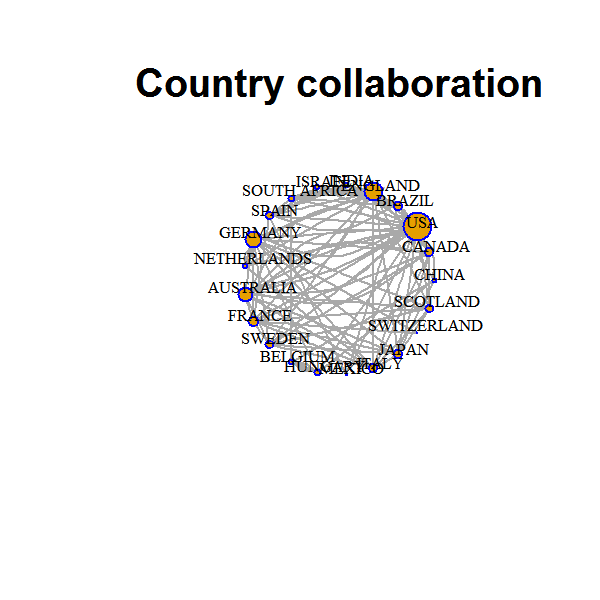### Co-Citation Network

# Create a co-citation network

NetMatrix <- biblioNetwork(M, analysis = "co-citation", network = "references", sep = ".  ")

# define functions from package Matrix
diag <- Matrix::diag
colSums <-Matrix::colSums

ind=which(Matrix::colSums(NetMatrix)-Matrix::diag(NetMatrix)>0)
NET=NetMatrix[ind,ind]

# Select number of vertices to plot
n <- 10    # n. of vertices
NetDegree <- sort(diag(NET),decreasing=TRUE)[n]
NET <- NET[diag(NET)>=NetDegree,diag(NET)>=NetDegree]

# delete diagonal elements (self-loops)
diag(NET) <- 0

# Create igraph object

# Remove loops
bsk.network <- simplify(bsk.network, remove.multiple = F, remove.loops = T)

# Choose Network layout
l = layout.fruchterman.reingold(bsk.network)

## Plot
plot(bsk.network,layout = l, vertex.label.dist = 0.5, vertex.frame.color = 'blue', vertex.label.color = 'black', vertex.label.font = 1, vertex.label = V(bsk.network)$name, vertex.label.cex = 0.5, main="Co-citation network")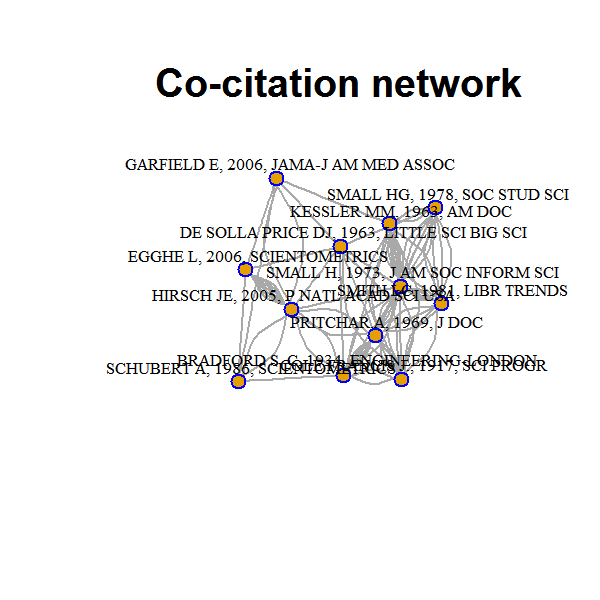### Keyword Coupling # Create a co-citation network NetMatrix <- biblioNetwork(M, analysis = "coupling", network = "keywords", sep = ";") # define functions from package Matrix diag <- Matrix::diag colSums <-Matrix::colSums # delete not linked vertices ind=which(Matrix::colSums(NetMatrix)-Matrix::diag(NetMatrix)>0) NET=NetMatrix[ind,ind] # Select number of vertices to plot n <- 10 # n. of vertices NetDegree <- sort(diag(NET),decreasing=TRUE)[n] NET <- NET[diag(NET)>=NetDegree,diag(NET)>=NetDegree] # delete diagonal elements (self-loops) diag(NET) <- 0 # Plot Keywords' Heatmap (most frequent 30 words) n=30 NETMAP=NetMatrix[ind,ind] MapDegree <- sort(diag(NETMAP),decreasing=TRUE)[n] NETMAP <- NETMAP[diag(NETMAP)>=MapDegree,diag(NETMAP)>=MapDegree] diag(NETMAP) <- 0 H <- heatmap(max(NETMAP)-as.matrix(NETMAP),symm=T, cexRow=0.3,cexCol=0.3)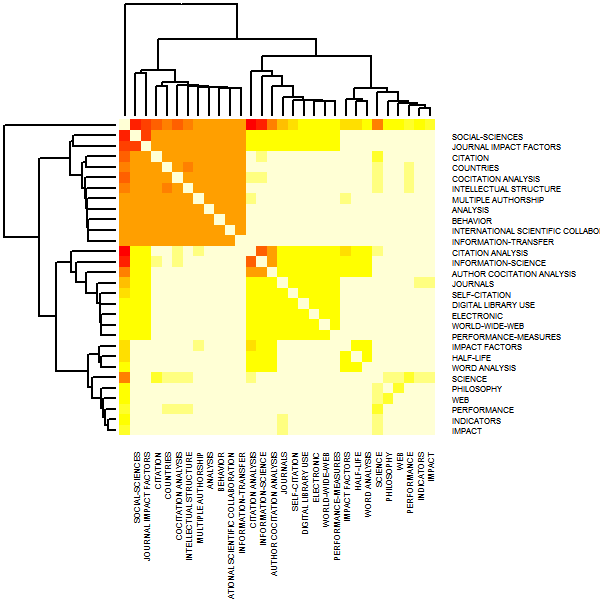# Create igraph object bsk.network <- graph.adjacency(NET,mode="undirected") # Remove loops bsk.network <- simplify(bsk.network, remove.multiple = T, remove.loops = T) # Choose Network layout l = layout.fruchterman.reingold(bsk.network) ## Plot plot(bsk.network,layout = l, vertex.label.dist = 0.5, vertex.frame.color = 'black', vertex.label.color = 'black', vertex.label.font = 1, vertex.label = V(bsk.network)$name, vertex.label.cex = 0.5, main="Keyword coupling")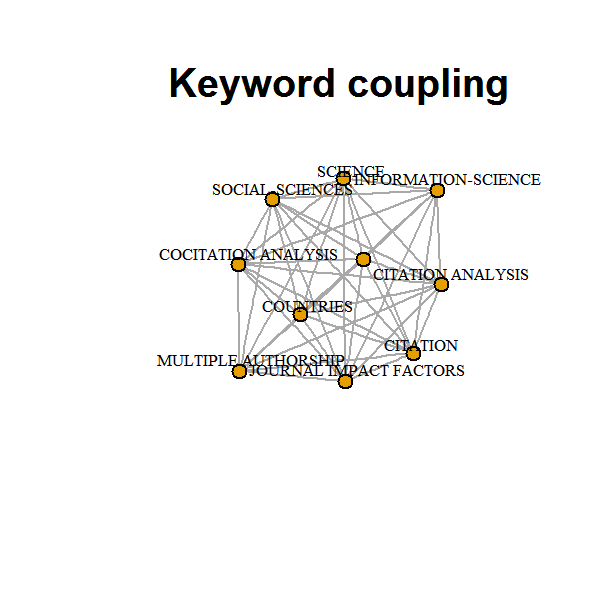## Co-Word Analysis: Conceptual structure of a field

The aim of the co-word analysis is to map the conceptual structure of a framework using the word co-occurrences in a bibliographic collection.

The analysis can be performed through dimensionality reduction techniques such as Multidimensional Scaling (MDS) or Multiple Correspondence Analysis (MCA).

In the following, we show an example using MCA to draw a conceptual structure of the field and K-means clustering to identify clusters of documents which express common concepts.

# Create a bipartite network of Keyword plus
#
# each row represents a manuscript
# each column represents a keyword (1 if present, 0 if absent in a document)

CW <- cocMatrix(M, Field = "ID", type="matrix", sep=";")

# dimension of CW
dim(CW)
##  300 489
# Define minimum degree (number of occurrences of each Keyword)
Degree=5
CW=CW[,colSums(CW)>=Degree]

# Delete empty rows
CW=CW[rowSums(CW)>0,]

# Dimension of Data matrix
dim(CW)
##  132  26
# Recode as dataframe
CW=data.frame(apply(CW,2,factor))

# Delete not consistent keywords
names(CW)
##   "INFORMATION"         "SCIENCE"             "H.INDEX"
##   "CITATION.ANALYSIS"   "V5"                  "INDICATORS"
##   "JOURNALS"            "PRODUCTIVITY"        "IMPACT.FACTOR"
##  "IMPACT.FACTORS"      "WEB"                 "TRENDS"
##  "PATTERNS"            "INDEX"               "IMPACT"
##  "ARTICLES"            "PERFORMANCE"         "PUBLICATION"
##  "INFORMATION.SCIENCE" "CITATION"            "SCOPUS"
##  "SELF.CITATION"       "SOCIAL.SCIENCES"
CW=CW[,-5]

# install and load FactoMineR and factoextra packages
if (!require("FactoMineR")){install.packages("FactoMineR")}
## Loading required package: FactoMineR
library(FactoMineR)
if (!require("factoextra")){install.packages("factoextra")}
## Loading required package: factoextra
## Loading required package: ggplot2
library(factoextra)

# Perform Multiple Correspondence Analysis (MCA)
res.mca <- MCA(CW, ncp=2, graph=FALSE)

# Get coordinates of keywords (we take only categories "1"")
coord=get_mca_var(res.mca)
df=data.frame(coord$coord)[seq(2,dim(coord$coord),by=2),]
row.names(df)=gsub("_1","",row.names(df))

# K-means clustering

# Selection of optimal number of clusters (silhouette method)
fviz_nbclust(scale(df), kmeans, method = "silhouette")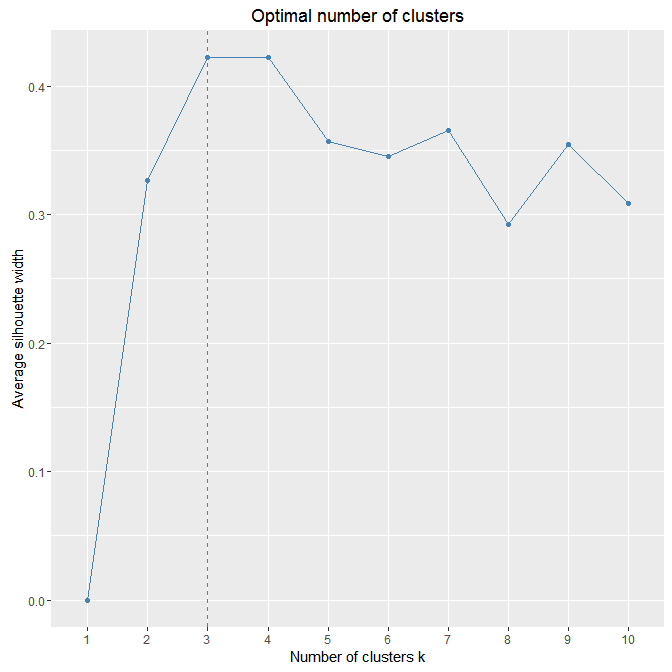# Partitions with 3 o 4 cluster are equally satisfactory. We prefer the solution with 4 clusters

# Perform the K-means clustering
km.res <- kmeans(scale(df), 4, nstart = 25)

# Plot of the conceptual map
fviz_cluster(km.res, data = df,labelsize=2)+theme_minimal()+
scale_color_manual(values = c("#00AFBB","#2E9FDF", "#E7B800", "#FC4E07"))+
scale_fill_manual(values = c("#00AFBB","#2E9FDF", "#E7B800", "#FC4E07")) +
labs(title= "     ") +
geom_point()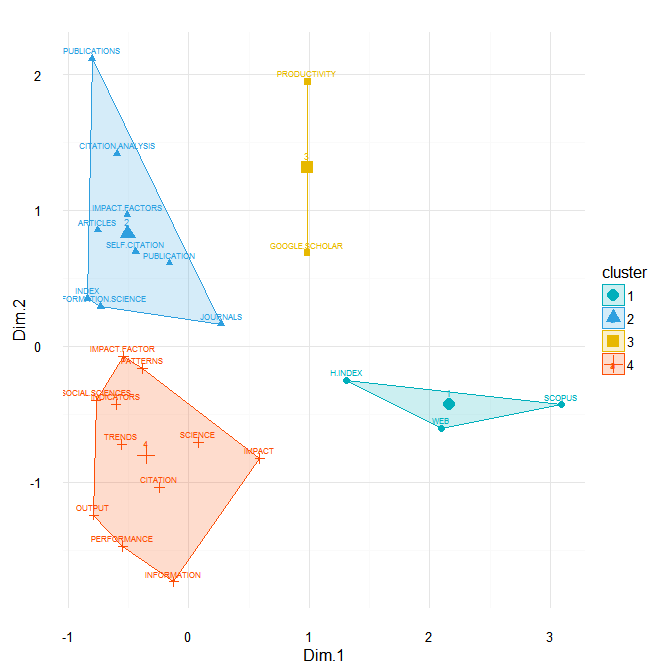## Historical Co-Citation Network

Historiographic map is a graph proposed by E. Garfield to represent a chronological network map of most relevant co-citations resulting from a bibliographic collection.

The function generates a chronological co-citation network matrix which can be plotted using “igraph”:

# Create a historical co-citation network

histResults <- histNetwork(M, n = 15, sep = ".  ")

# Create igraph object
plot(bsk.network,layout = l, vertex.label.dist = 0.5, vertex.frame.color = 'blue', vertex.label.color = 'black', vertex.label.font = 1, vertex.label = row.names(histResults[]), vertex.label.cex = 0.4, edge.arrow.size=0.1)